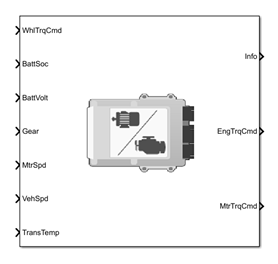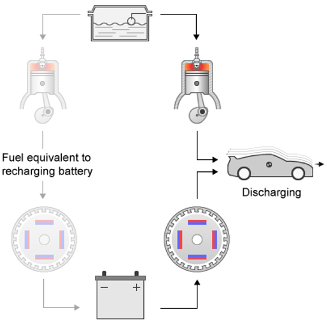# Equivalent Consumption Minimization Strategy

Energy management controller for P0–P4 hybrid electric vehicles

• Library:
• Powertrain Blockset / Propulsion / Supervisory Controllers

•## Description

Use the Equivalent Consumption Minimization Strategy (ECMS) block to control the energy management of hybrid electric vehicles (HEVs). The block optimizes the torque split between the engine and motor to minimize energy consumption while maintaining the battery state of charge (SOC).

The HEV P0, P1, P2, P3, and P4 reference applications use the Equivalent Consumption Minimization Strategy block for hybrid control.

Use the Motor location parameter to specify the HEV motor location.Use the ECMS method parameter to implement either an adaptive or non-adaptive ECMS method. The HEV architectures are charge-sustaining, meaning the battery SOC must remain in a specified range because there is no plugin capability to recharge the battery. The battery is an energy buffer, and all energy comes from the fuel if the change in SOC is minimized over a drive cycle. To sustain the charge over a specified drive cycle, the block implements either of these ECMS methods.

ECMS MethodDescription

`Non-adaptive` (default)

The block uses a constant ECMS equivalence factor.

• Use this method to determine the best fuel economy over a drive cycle.

• If you change the drive cycle or HEV architecture, retune the ECMS weighting factor to maintain the ending SOC.

• By default, the block uses a single constant.

`Adaptive`

The block adjusts an ECMS equivalence factor by using the output of a PI controller.

• Use this method to maintain the SOC and minimize the delta SOC over many drive cycles. The block:

• Tunes the PI controller gains.

• Sustains the SOC.

• The PI controller minimizes the error between the target SOC and current SOC.

### ECMS Control Algorithm

The block implements a dynamic supervisory controller that determines the engine torque, motor torque, starter, clutch, and brake pressure commands. Specifically, the block:

• Converts the driver accelerator pedal signal to a wheel torque request. To calculate the total powertrain torque at the wheels, the algorithm uses the maximum engine torque and motor torque curves and the transmission and differential gear ratios.

• Converts the driver brake pedal signal to a brake pressure request. The algorithm multiplies the brake pedal signal by a maximum brake pressure.

• Implements a regenerative braking algorithm for the traction motor to recover the maximum amount of kinetic energy from the vehicle.

The block implements an ECMS algorithm that optimizes the torque split between the engine and motor to minimize energy consumption while maintaining the battery SOC. Specifically, the ECMS:

• Assigns a cost to electrical energy, so that using stored electrical energy is equal to consuming fuel energy.

Battery ModeEquivalent Electrical EnergyDescription

Discharging

Positive

Battery discharges stored electrical energy when the electric machine is in use.Charging

Negative

Battery stores electrical energy from either the:

• Engine and electric machine acting as a generator

• Electric machine acting as a generator during regenerative braking• Is an instantaneous minimization method that the software solves at every controller time step. To implement the strategy, the ECMS selects the optimal motor and engine torque in the optimization strategy to minimize the equivalent energy consumption.

## Ports

### Input

expand all

Wheel torque command.

Data Types: `double`

Battery state of charge.

Data Types: `double`

Battery voltage.

Data Types: `double`

Transmission gear.

Data Types: `double`

Motor speed.

Data Types: `double`

Vehicle speed, in m/s.

Data Types: `double`

Transmission temperature, in K.

Data Types: `double`

### Output

expand all

Block data, returned as a bus signal that contains these block values.

Signal DescriptionUnits

`EngTrqCmd`

Engine torque command

N·m

`MtrTrqCmd`

Motor torque command

N·m

`EquivFctr`

Equivalence factor

NA

`MinHamil`

Minimum Hamiltonian

kW

Engine torque command, in N·m.

Data Types: `double`

Motor torque command, in N·m.

Data Types: `double`

## Parameters

expand all

Block Options

Specify the HEV motor location.Use the ECMS method parameter to implement either an adaptive or non-adaptive ECMS method. The HEV architectures are charge-sustaining, meaning the battery SOC must remain in a specified range because there is no plugin capability to recharge the battery. The battery is an energy buffer, and all energy comes from the fuel if the change in SOC is minimized over a drive cycle. To sustain the charge over a specified drive cycle, the block implements either of these ECMS methods.

ECMS MethodDescription

`Non-adaptive` (default)

The block uses a constant ECMS equivalence factor.

• Use this method to determine the best fuel economy over a drive cycle.

• If you change the drive cycle or HEV architecture, retune the ECMS weighting factor to maintain the ending SOC.

• By default, the block uses a single constant.

`Adaptive`

The block adjusts an ECMS equivalence factor by using the output of a PI controller.

• Use this method to maintain the SOC and minimize the delta SOC over many drive cycles. The block:

• Tunes the PI controller gains.

• Sustains the SOC.

• The PI controller minimizes the error between the target SOC and current SOC.

Differential

Differential gear ratio. No dimension.

Data Types: `double`

Differential efficiency factor. No dimension.

Data Types: `double`

Data Types: `double`

Transmission

Transmission efficiency factors.

Data Types: `double`

Transmission gear number vector. No dimension.

Data Types: `double`

Transmission gear ratio vector. No dimension.

Data Types: `double`

Transmission efficiency vector. No dimension.

#### Dependencies

To enable this parameter, set Transmission efficiency factors to `Gear only`.

Data Types: `double`

Transmission efficiency torque breakpoints, in N·m.

#### Dependencies

To enable this parameter, set Transmission efficiency factors to `Gear, input torque, input speed, and temperature`.

Data Types: `double`

Transmission efficiency speed breakpoints, in rad/s.

#### Dependencies

To enable this parameter, set Transmission efficiency factors to `Gear, input torque, input speed, and temperature`.

Data Types: `double`

Transmission efficiency temperature breakpoints, in K.

#### Dependencies

To enable this parameter, set Transmission efficiency factors to `Gear, input torque, input speed, and temperature`.

Data Types: `double`

Transmission efficiency vector. No dimension.

#### Dependencies

To enable this parameter, set Transmission efficiency factors to `Gear, input torque, input speed, and temperature`.

Data Types: `double`

Engine

Speed breakpoints, in rpm.

Data Types: `double`

Commanded torque breakpoints, in N·m.

Data Types: `double`

Brake torque map, in N·m.

Data Types: `double`

Minimum engine torque command table, in N·m.

Data Types: `double`

Fuel flow map, in kg/s.

Data Types: `double`

Minimum engine torque command, in N·m.

Data Types: `double`

Fuel lower heating value, in J/kg.

Data Types: `double`

Engine idle speed, in rpm.

Data Types: `double`

Battery

Battery state-of-charge breakpoints. No dimension.

Data Types: `double`

Battery charge limit table. No dimension.

Data Types: `double`

Battery discharge limit table. No dimension.

Data Types: `double`

Maximum battery current, in A.

Data Types: `double`

DC/DC converter efficiency. No dimension.

Data Types: `double`

Maximum battery charge power, in W.

Data Types: `double`

Maximum battery discharge power, in W.

Data Types: `double`

Motor

Motor maximum torque table, in N·m.

Data Types: `double`

Motor speed breakpoints, in rpm.

Data Types: `double`

Motor torque breakpoints, in N·m.

Data Types: `double`

Motor efficiency map. No dimension.

Data Types: `double`

Number of motor torque calculation points. No dimension.

Data Types: `double`

P0 belt ratio. No dimension.

#### Dependencies

To enable this parameter, set Motor location to `P0`.

Data Types: `double`

Energy Management

ECMS weighting factor. No dimension.

Data Types: `double`

Penalty factor power. No dimension.

Data Types: `double`

Adaptive ECMS proportional gain. No dimension.

#### Dependencies

To enable this parameter, set ECMS method to `Adaptive`.

Data Types: `double`

Adaptive ECMS integral gain. No dimension.

#### Dependencies

To enable this parameter, set ECMS method to `Adaptive`.

Data Types: `double`

Constraint penalty factor. No dimension.

Data Types: `double`

Target battery state-of-charge. No dimension.

Data Types: `double`

Minimum battery state-of-charge. No dimension.

Data Types: `double`

Maximum battery state-of-charge. No dimension.

Data Types: `double`

### Acknowledgments

MathWorks® would like to acknowledge the contribution of Dr. Simona Onori to the ECMS optimal control algorithm implemented in this block. Dr. Onori is a Professor of Energy Resources Engineering at Stanford University. Her research interests include electrochemical modeling, estimation and optimization of energy storage devices for automotive and grid-level applications, hybrid and electric vehicles modeling and control, PDE modeling, and model-order reduction and estimation of emission mitigation systems. She is a senior member of IEEE®.

 Balazs, A., Morra, E., and Pischinger, S., Optimization of Electrified Powertrains for City Cars. SAE Technical Paper 2011-01-2451. Warrendale, PA: SAE International Journal of Alternative Powertrains, 2012.

 Onori, S., Serrao, L., and Rizzoni, G., Hybrid Electric Vehicles Energy Management Systems. New York: Springer, 2016.

## SupportGet trial now